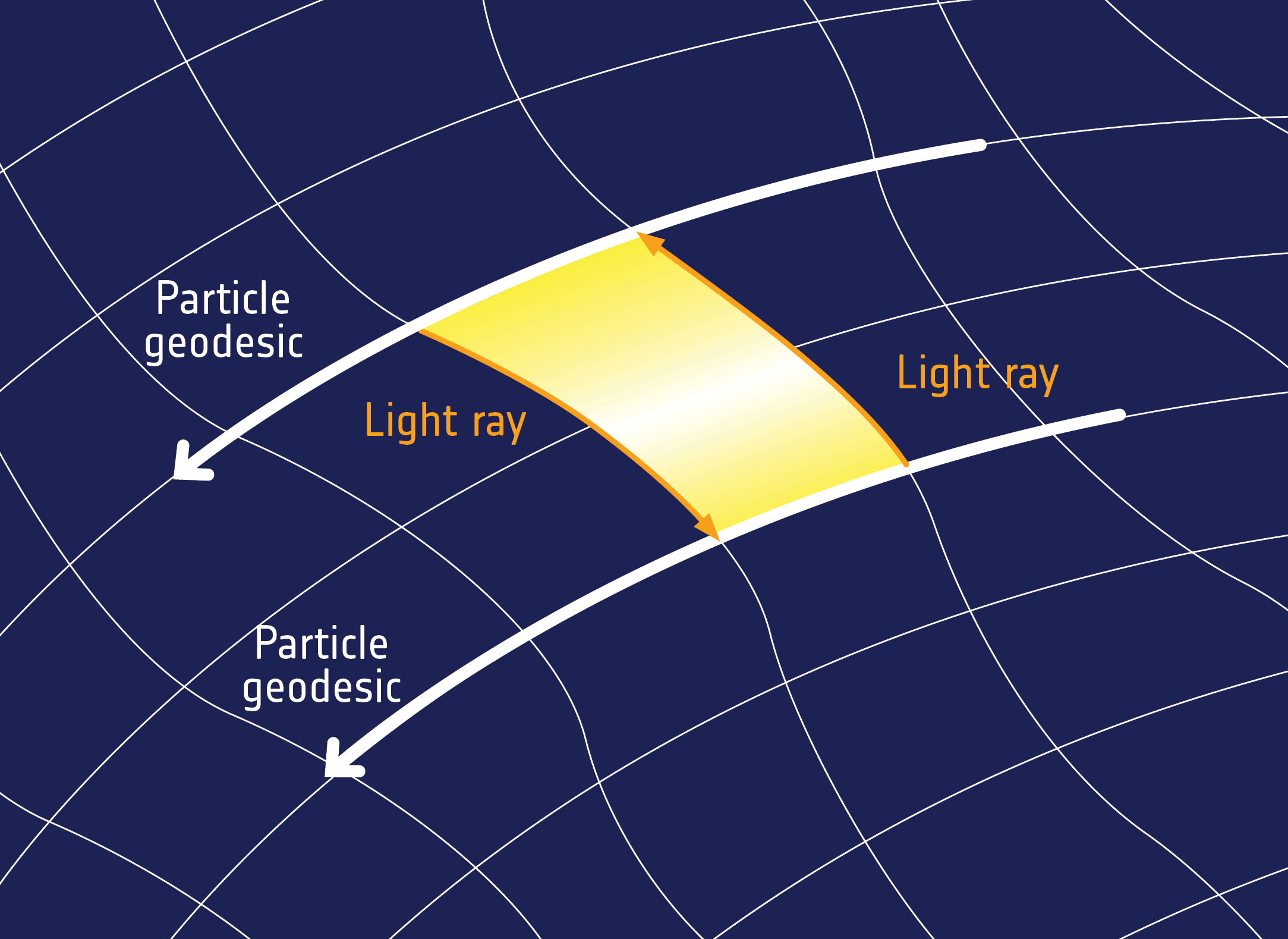# I Have Some Questions About Spacetime And Gravity

Gravity spacetime

2 replies to this topic

### #1Lucqs

Lucqs

Curious

•• Members
•• 1 posts

Posted 04 November 2017 - 04:32 PM

I'm gonna start off by saying that I by no means am an expert on physics.

I'm only 14, but I'm curious about spacetime and gravity.

My current understanding is that time actually is bent in a curve relative to mass, and that we perceivee time as being straight but that it actually is bent because straight lines cant exist in a universe filled with mass.

Im pretty sure Im missing something, so to what extent is this correct?

Thanks

Edited by Lucqs, 04 November 2017 - 04:34 PM.

### #2exchemist

exchemist

Creating

•• Members
•• 2914 posts

Posted 05 November 2017 - 04:33 AM

I'm gonna start off by saying that I by no means am an expert on physics.

I'm only 14, but I'm curious about spacetime and gravity.

My current understanding is that time actually is bent in a curve relative to mass, and that we perceivee time as being straight but that it actually is bent because straight lines cant exist in a universe filled with mass.

Im pretty sure Im missing something, so to what extent is this correct?

Thanks

Join the club! I'm not an expert on it either.

I believe what you may have in mind is the idea of "geodesics" in general relativity. A geodesic is the equivalent of a straight line, but in curved geometries, the original geodesic being the shortest distance between two points on the surface of a sphere, such as the Earth. The surface of a sphere is 2D but not flat (e.g. the 3 angles of a triangle do not any longer add up to 180deg, as you can check by drawing a triangle on an orange) so the geometry does not obey Euclid's rules which is what we learn at school. My understanding is that the path , called the "worldline", of something in the 4D spacetime geometry of GR follows a geodesic in that 4D geometry, and that that geometry is made to curve by mass

So it is not that time is somehow curved but 4D spacetime is curved - by mass - and then objects follow geodesics (the equivalents of straight lines)  in that curved spacetime.

Bear in mind that the worldline of even a stationary object is a line, not a point, because this is spacetime and so if it exists for a finite period of time it will follow a line in the time dimension, even though it does not move along any of the space dimensions.

Probably clear as mud, but that's the best I can do.Perhaps someone else can add to this without confusing you......

Edited by exchemist, 05 November 2017 - 04:38 AM.

• sanctus likes this

### #3Vmedvil

Vmedvil

Understanding

•• Members
•• 370 posts

Posted 06 November 2017 - 02:54 PM

Actually, that is correct

I'm gonna start off by saying that I by no means am an expert on physics.

I'm only 14, but I'm curious about spacetime and gravity.

My current understanding is that time actually is bent in a curve relative to mass, and that we perceivee time as being straight but that it actually is bent because straight lines cant exist in a universe filled with mass.

Im pretty sure Im missing something, so to what extent is this correct?

Thanks

Yes, the Curvature of space-time is governed by Special Relativity and General Relativity it is not so much mass that curves time-space as energy which mass is just a compressed form of energy. Time and Space are interconnected in a 3+1 dimensional fabric, the points of space are actually bend toward the higher density of energy due to length's actually being shorter in those regions due to length contraction which time is longer in these volumes as well the length of the cycles of time run more slowly. Even if you move at a high velocity you gain more Energy and begin to create this same effect. The shortest distance between two points is a geodesic which Exchemist explained well. Gravity is actually a curvature caused by the presence of energy in a volume being more compressed energy mass has a larger effect than just energy. The curvature goes into a hyperspace domain being the +1 dimension. The particle will always follow the geodesic being the shortest length between two points.It is visualized in 2 + 1 dimensions to make it easier to understand as flat like this image and the next one.But actually it is in reality 3+1 manifesting itself like this.